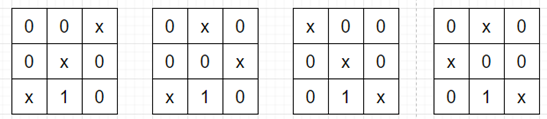# Program to check how many ways we can choose empty cells of a matrix in python

Suppose we have a N x N binary matrix where 0 is for empty cells and 1 is a blocked cells, we have to find the number of ways to choose N empty cells such that every row and every column has at least one chosen cells. If the answer is very large return result mod 10^9 + 7

So, if the input is like

 0 0 0 0 0 0 0 1 0

then the output will be 4, as we have following configurations (where x is a selected cell) −To solve this, we will follow these steps −

• n := size of matrix
• Define a function f() . This will take i, bs
• if i >= n, then
• return 1
• ans := 0
• for j in range 0 to n, do
• if matrix[i, j] is same as 0 and (2^j AND bs is same as 0) , then
• ans := ans + f(i + 1, bs OR 2^j)
• return ans
• From the main method call and return f(0, 0)

Let us see the following implementation to get better understanding −

## Example

Live Demo

class Solution:
def solve(self, matrix):
n = len(matrix)

def f(i, bs):
if i >= n:
return 1
ans = 0
for j in range(n):
if matrix[i][j] == 0 and ((1 << j) & bs == 0):
ans += f(i + 1, bs | (1 << j))
return ans

return f(0, 0)

ob = Solution()
matrix = [
[0, 0, 0],
[0, 0, 0],
[0, 1, 0]
]
print(ob.solve(matrix))

## Input

[
[0, 0, 0],
[0, 0, 0],
[0, 1, 0] ]

## Output

4